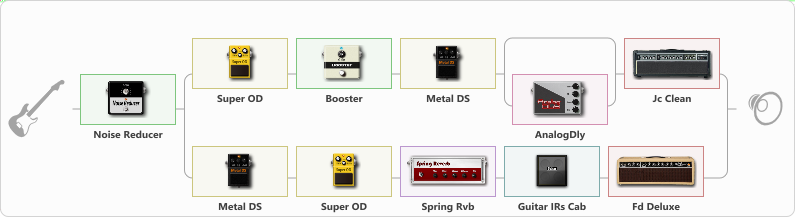# An4

Discussion in 'ToneLib-GFX presets' started by Anulu, Nov 21, 2020.

1. An4

Preset name: An4

Effects chain:Effect: "Noise Reducer" (Dynamics / Filter), active - "yes"
{
"Sens" = 85
"Mode" = Soft
}

Effect: "Splitter" (Dynamics / Filter), active - "yes"
{
"A-Bypass" = Off
"A-Pan" = 0
"A-Level" = 55
"B-Bypass" = Off
"B-Pan" = 0
"B-Level" = 55

'A' branch:
{

Effect: "Super OD" (Overdrive / Distortion), active - "yes"
{
"Drive" = 8
"Tone" = 80
"Level" = 91
}

Effect: "Booster" (Dynamics / Filter), active - "yes"
{
"Gain" = 52
}

Effect: "Metal DS" (Overdrive / Distortion), active - "yes"
{
"Dist" = 100
"Bass" = 74
"Middle" = 60
"Treble" = 46
"Level" = 57
}

Effect: "Splitter" (Dynamics / Filter), active - "yes"
{
"A-Bypass" = Off
"A-Pan" = 0
"A-Level" = 55
"B-Bypass" = Off
"B-Pan" = 0
"B-Level" = 55

'A' branch:
{
}
'B' branch:
{

Effect: "AnalogDly" (Delay), active - "yes"
{
"Time" = 380
"Feedback" = 34
"Tone" = 65
"Mix" = 65
}
}
}

Effect: "Jc Clean" (Amp simulators), active - "yes"
{
"Gain" = 60
"Bass" = 50
"Middle" = 50
"Treble" = 50
"Presence" = 58
"Master" = 50
"Level (dB)" = 2
}
}
'B' branch:
{

Effect: "Metal DS" (Overdrive / Distortion), active - "yes"
{
"Dist" = 100
"Bass" = 74
"Middle" = 60
"Treble" = 46
"Level" = 57
}

Effect: "Super OD" (Overdrive / Distortion), active - "yes"
{
"Drive" = 8
"Tone" = 80
"Level" = 91
}

Effect: "Spring Rvb" (Reverberation), active - "yes"
{
"Time" = 7.0
"PreDelay" = 0
"LoDamp" = 16
"HiDamp" = 26
"Mix" = 42
}

Effect: "Guitar IRs Cab" (Cabinets), active - "yes"
{
"Model" = Peavey 5150 (4x12")
"Mic Position" = Middle
"Mic Distance" = Middle
"Low Cut (Hz)" = 85
"Hi Cut (kHz)" = 18.5
"Mix" = 100
"Level (dB)" = 3
}

Effect: "Fd Deluxe" (Amp simulators), active - "yes"
{
"Gain" = 85
"Bass" = 50
"Middle" = 50
"Treble" = 50
"Presence" = 56
"Master" = 50
"Level (dB)" = 0
}
}
}

Note: You will need to download and install the ToneLib-GFX software to use the preset.

File size:
1.1 KB
Views:
2,692